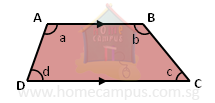## Four-sided Figures: Types and Properties

Practice Unlimited Questions

#### FOUR-SIDED FIGURES

This lesson covers the following four-sided figures:

#### 1. RectanglesIn a rectangle:
• opposite sides are parallel and equal
• all angles are 90°

#### 2. SquaresIn a square:
• all sides are equal
• opposite sides are parallel
• all angles are 90°

#### 3. ParallelogramsIn a parallelogram:
• opposite sides are parallel and equal
Rectangles and squares are examples of parallelograms.

#### 4. RhombusesIn a rhombus:
• all sides are equal
• opposite sides are parallel
A square is an example of a rhombus.

#### 5. Trapeziums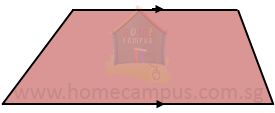A trapezium has:
• one pair of parallel sides

#### 6. Opposite angles of a parallelogram

Make a parallelogram or trapezium using a set square and a ruler, or trace one from your book. Now, measure its angles using a protractor. What do you notice?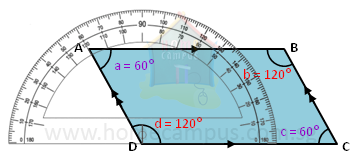In our case,
∠a  =  60°
∠b  =  120°
∠c  =  60°
∠d  =  120°

Therefore,
∠a  =  ∠c
∠b  =  ∠d
Conclusion: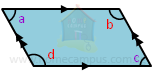∠a = ∠c
∠b = ∠d
The opposite angles of a parallelogram are equal.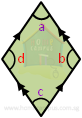∠a = ∠c
∠b = ∠d
The opposite angles of a rhombus are equal (as a rhombus is a type of parallelogram).

#### 7. Sum of a pair of angles between two parallel lines

Make a parallelogram or trapezium using a set square and a ruler, or trace one from your book. Now, using a protractor, measure all the angles of the figure. What do you notice?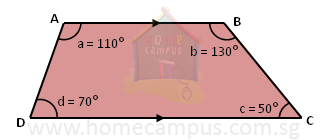In our case,
∠a  =  110°
∠b  =  130°
∠c  =  50°
∠d  =  70°

Therefore,
∠a  +  ∠d  =  110°  +  70°  =  180°
∠b  +  ∠c  =  130°  +  50°  =  180°
Conclusion:
The sum of a pair of angles between two parallel lines is 180°.

In a parallelogram,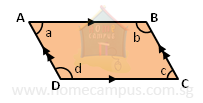AB // DC
So,
∠a + ∠d = 180°, and ∠b + ∠c = 180°